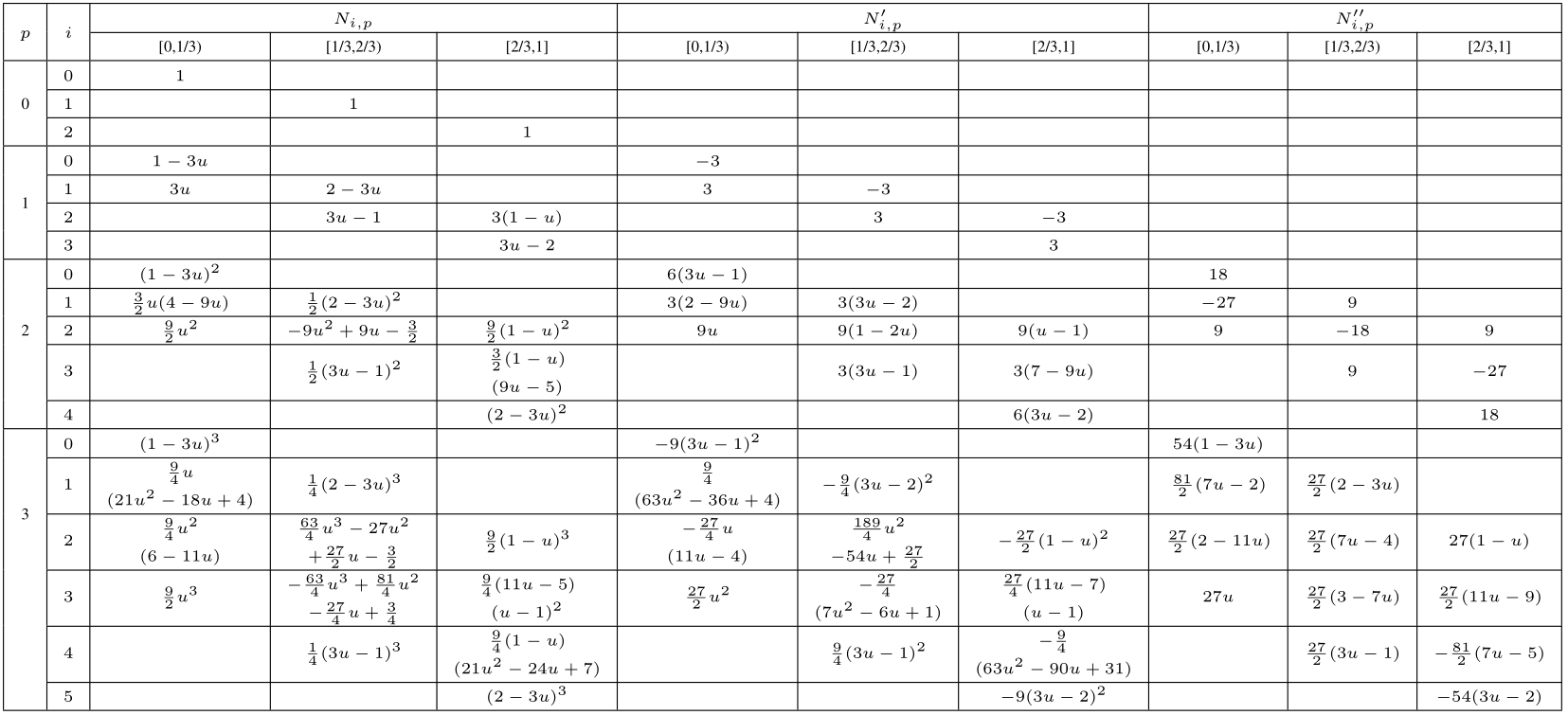### Definition

A pth-degree B-spline curve is defined by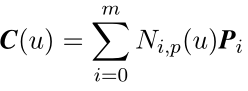where Pi are the control points, and Ni,p(u) are the pth-degree B-spline functions defined on the knot vector (m+1 knots):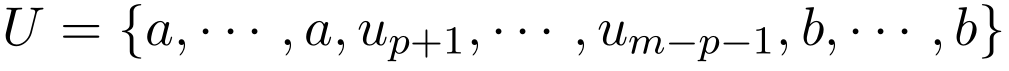U is a nondecreasing sequence of real numbers. The ui are called knots, and U is called the knot vector. The ith B-spline basis function of pth-degree, denoted by Ni,p(u), is defined as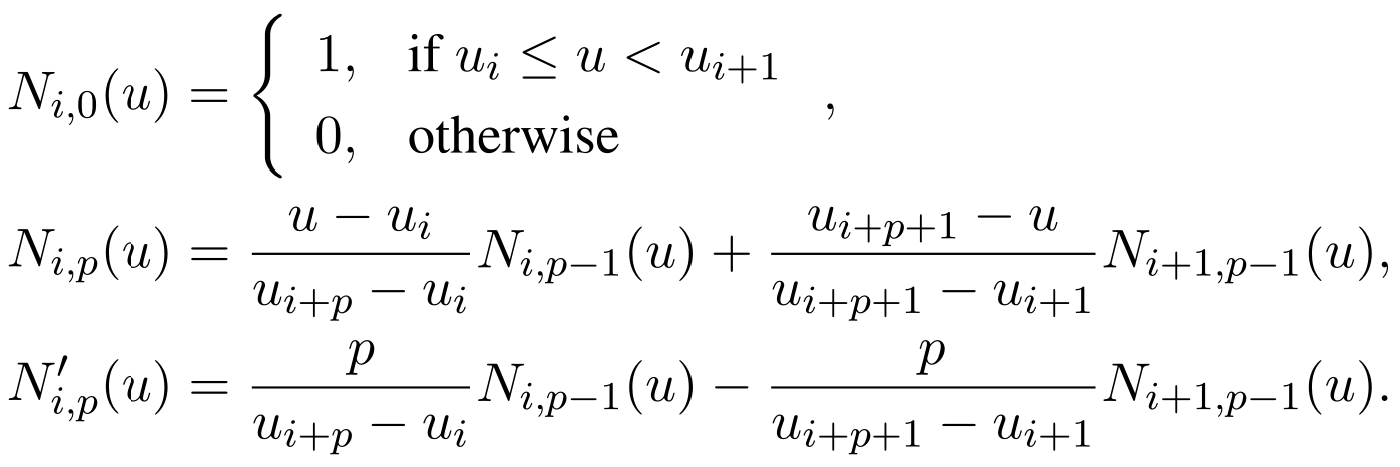Ni,0(u) is a step function which only equal to 1 at its own interval [ui, ui+1). And Ni,p(u) at higher degree is a linear combination of two (p−1)th-degree basis functions. The combination of step functions leads to their local support property. It should be noted that 0/0 is specially defined to be equal to 0 in the computation.

### Analytic Expression

The analytic expressions of Ni,p(u) when p=0, 1, 2, 3 are listed in the Table 123, and 4, respectively. The middle knots means that their knot value are neither 0 nor 1. Here the knot vector is defined with uniform intervals. And the graphs of Ni,p(u) are given in the Figure 123, and 4, respectively. In the graphs, the dashed vertical lines separate the different knot intervals. When we add the expressions in the same interval and same degree together, the summation will always be one, which is called one of their properties, partition of unity. According to the graphs of the basis functions, their important properties are illustrated, such as, local support property and nonnegativity.

Table 1: Analytic Expression of Ni,p(u) for 0 middle knot, Bézier function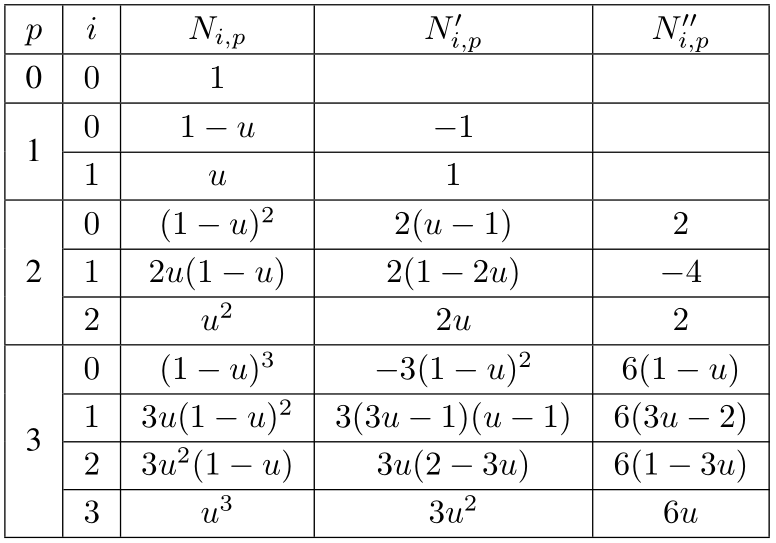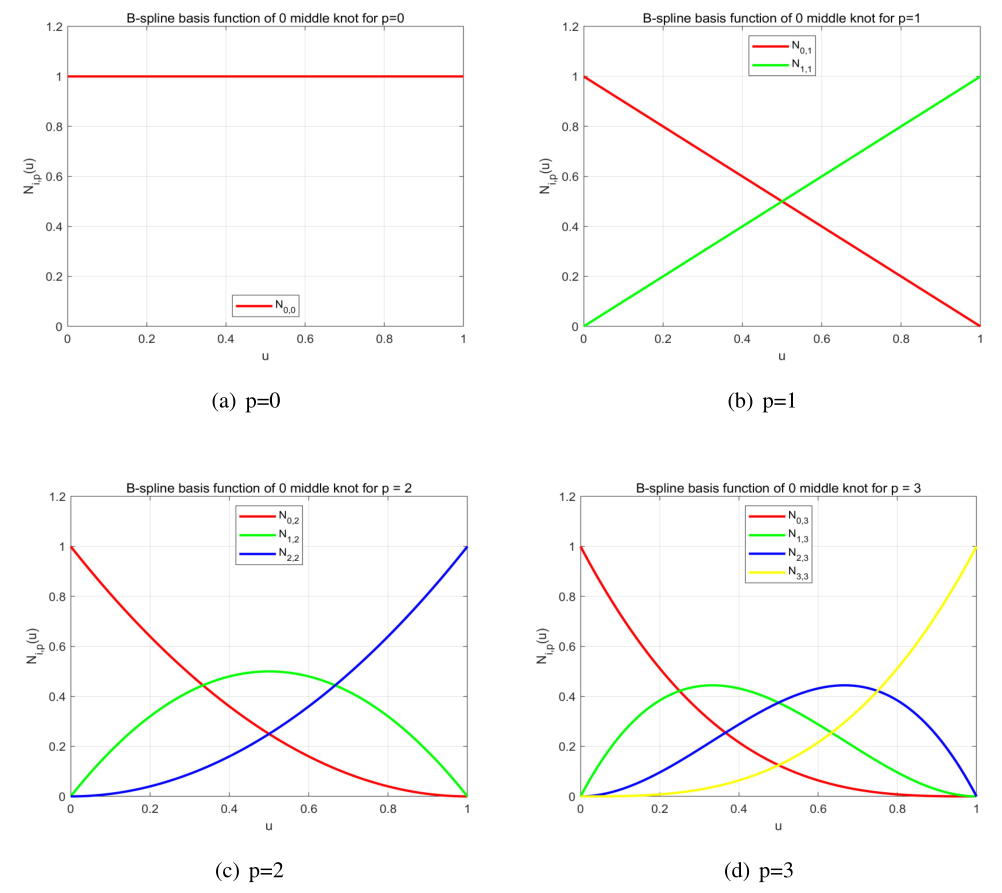Figure 1: Graph of Ni,p(u) for 0 middle knot

Table 2: Analytic Expression of Ni,p(u) for 1 middle knot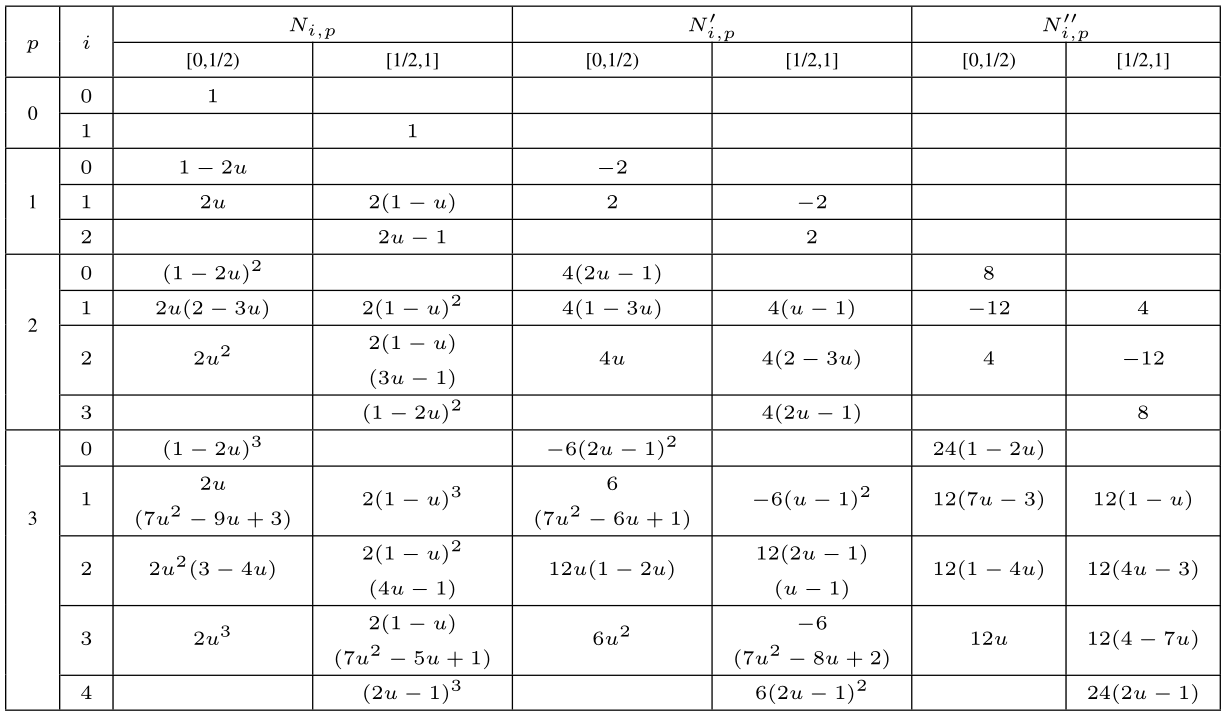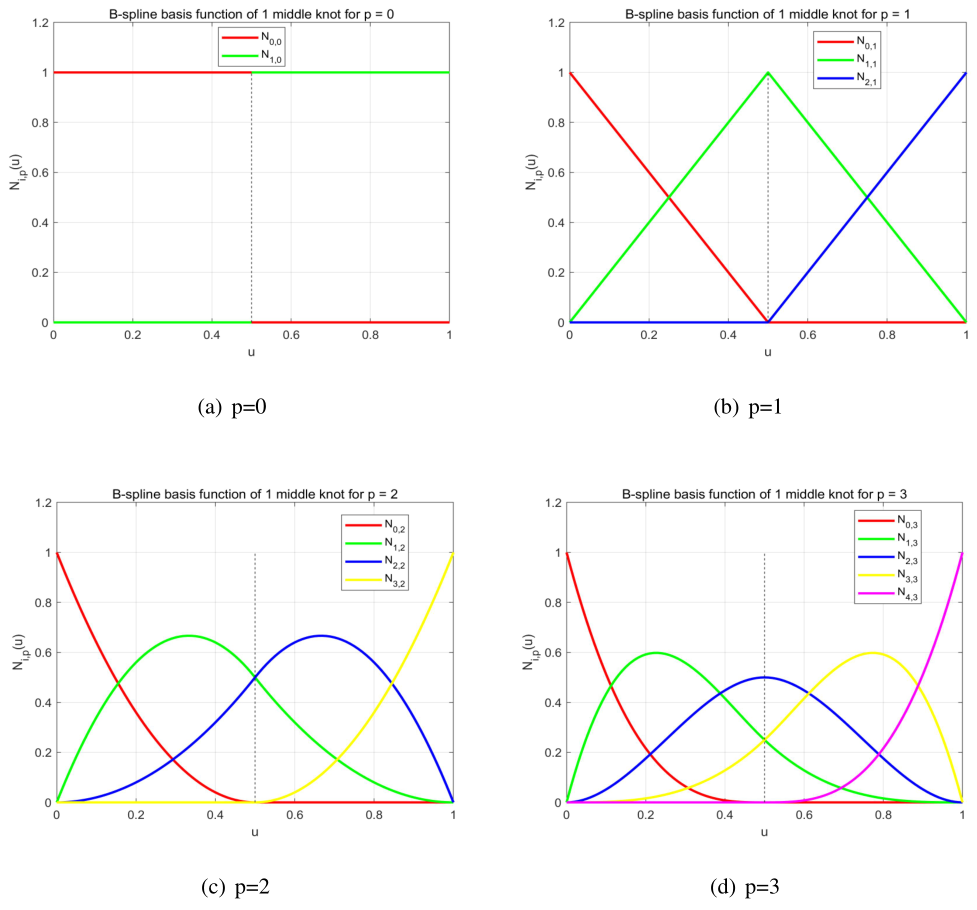Figure 2: Graph of Ni,p(u) for 1 middle knot

Table 3: Analytic Expression of Ni,p(u) for 2 middle knots